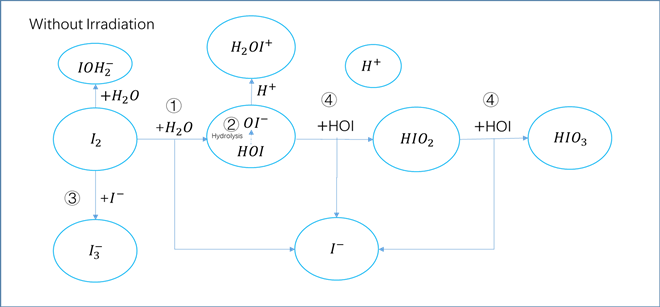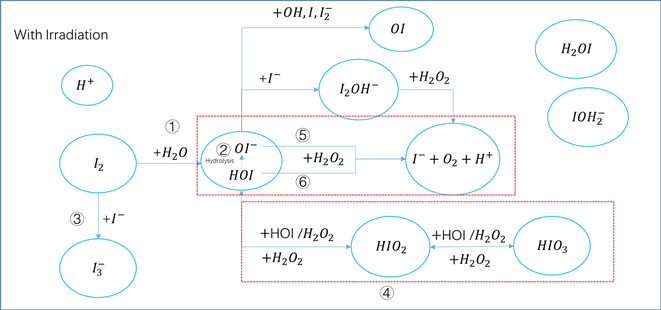﻿ 离子碘向元素碘转化行为模型研究

# 离子碘向元素碘转化行为模型研究Study on Models for the Conversion Behavior of Iodine Ions to Elemental Iodine in Aqueous Solutions

Abstract: Radiolytic conversion of iodine ions (I) to elemental iodine (I2) occurs in aqueous solutions under radiation, resulting in the escape of I2 from the solution. This phenomenon could influence the accident source term significantly so that it has to be considered seriously when performing accident source term analysis. In this paper, the iodine chemical model without irradiation condition and irradiation condition is summarized. The formula promotion and reaction rate constant in NUREG/CR-5950 of Nuclear Regulatory Commission of the United States are introduced, which can be used to support deterministic accident consequence analysis. Finally, a summary and suggestions for further research are given.

1. 引言

2. 无辐照条件下的碘化学模型

${I}_{2}+{H}_{2}O⇌{I}^{-}+HOI+{H}^{+}$ (1)

$HOI⇌O{I}^{-}+{H}^{+}$ (2)

${I}_{2}+{I}^{-}⇌{I}_{3}^{-}$ (3)

$HOI+HOI⇌{I}^{-}+HI{O}_{2}+{H}^{+}$ (4a)

$HOI+HI{O}_{2}⇌{I}^{-}+HI{O}_{3}+{H}^{+}$ (4b)

3. 辐照条件下的碘水化学模型

${\text{IO}}^{-}+{\text{H}}_{2}{\text{O}}_{2}⇌{\text{I}}^{-}+{\text{O}}_{2}+{\text{H}}_{2}\text{O}$ (5)

$\text{HOI}+{\text{H}}_{2}{\text{O}}_{2}⇌{\text{H}}^{+}+{\text{I}}^{-}+{\text{O}}_{2}+{\text{H}}_{2}\text{O}$ (6)

${I}^{-}+HOI⇌{I}_{2}O{H}^{-}$ (7a)

${I}_{2}O{H}^{-}+{H}_{2}{O}_{2}⇌2{\text{I}}^{-}+{\text{O}}_{2}+{H}^{+}+{H}_{2}O$ (7b)

${\text{IO}}_{3}^{-}+{H}_{2}{O}_{2}\to I{O}_{2}^{-}+{H}_{2}O+{O}_{2}$ (7c)

${\text{IO}}_{3}^{-}+{H}^{+}+{H}_{2}{O}_{2}\to HI{O}_{2}+{H}_{2}O+{O}_{2}$ (7d)

${\text{HIO}}_{2}+{H}_{2}{O}_{2}\to HIO+{O}_{2}+{H}_{2}O$ (7e)

${\text{HIO}}_{2}+{I}^{-}+{H}^{+}\to 2HIO+{H}_{2}O$ (7f)

$\text{HIO}+{I}^{-}+{H}^{+}⇌{I}_{2}+{H}_{2}O$ (7g)

${\text{IO}}_{3}^{-}+{H}_{2}{O}_{2}\to I{O}_{4}^{-}+{H}_{2}O$ (8a)

${\text{HIO}}_{2}+{H}_{2}{O}_{2}\to I{O}_{3}^{-}+{H}^{+}+{H}_{2}O$ (8b)

$\text{OH}+\text{HOI}\to \text{IO}+{\text{H}}_{2}\text{O}$ (8c)

$\text{I}+\text{HOI}\to \text{IO}+{\text{I}}^{-}+{\text{H}}^{+}$ (8d)

${\text{I}}_{2}^{-}+\text{HOI}\to \text{IO}+2{\text{I}}^{-}+{\text{H}}^{+}$ (8e)Figure 1. Diagram of iodine compounds in solution without irradiationFigure 2. Diagram of iodine compounds in solution under irradiation condition

4. NUREG/CR-5950中推荐的模型及反应率常数

Abel  提出了在辐照环境下，I、I2和H+之间存在以下关系式，：

$\frac{{\left[{\text{H}}^{+}\right]}^{2}×{\left[{\text{I}}^{-}\right]}^{2}}{\left[{\text{I}}_{\text{2}}\right]}=a+b×\left[{\text{H}}^{+}\right]$ (9)

$\left[{H}^{+}\right]={10}^{-pH}$ (10)

① 反应率描述方程

${\text{I}}^{-}+{\text{H}}_{2}{\text{O}}_{2}\to {\text{IO}}^{-}+{\text{H}}_{2}\text{O}$ (11)

${r}_{1}={k}_{1}×\left[{\text{I}}^{-}\right]×\left[{\text{H}}_{\text{2}}{\text{O}}_{\text{2}}\right]$ (12)

${\text{IO}}^{-}+{\text{H}}_{2}{\text{O}}_{2}\stackrel{{k}_{5}}{⇄}{\text{I}}^{-}+{\text{O}}_{2}+{\text{H}}_{2}\text{O}$ (13)

$\text{HOI}+{\text{H}}_{2}{\text{O}}_{2}\stackrel{{k}_{6}}{⇄}{\text{H}}^{+}+{\text{I}}^{-}+{\text{O}}_{2}+{\text{H}}_{2}\text{O}$ (14)

${r}_{2}={k}_{6}×\left[\text{HIO}\right]×\left[{\text{H}}_{2}{\text{O}}_{2}\right]+{k}_{5}×\left[{\text{IO}}^{-}\right]×\left[{\text{H}}_{2}{\text{O}}_{2}\right]$ (15)

② 稳定状态意味着氧化反应和还原反应的反应率相同，即 ${r}_{1}={r}_{2}$，所以有：

${k}_{1}×\left[{\text{I}}^{-}\right]×\left[{\text{H}}_{2}{\text{O}}_{2}\right]={k}_{6}×\left[\text{HIO}\right]×\left[{\text{H}}_{2}{\text{O}}_{2}\right]+{k}_{5}×\left[{\text{IO}}^{-}\right]×\left[{\text{H}}_{2}{\text{O}}_{2}\right]$ (16)

${k}_{1}\left[{\text{I}}^{-}\right]={k}_{6}\left[\text{HIO}\right]+{k}_{5}\left[{\text{IO}}^{-}\right]$ (17)

③ 次碘酸浓度的推导

$\left[\text{HIO}\right]={K}_{1}×\frac{{\text{I}}_{2}}{\left[{\text{H}}^{+}\right]×\left[{\text{I}}^{-}\right]}$ (18)

$\left[{\text{IO}}^{-}\right]=\frac{{K}_{2}×\left[\text{HIO}\right]}{\left[{\text{H}}^{+}\right]}=\frac{{K}_{2}×{K}_{1}×\left[{\text{I}}_{2}\right]}{{\left[{\text{H}}^{+}\right]}^{2}×\left[{\text{I}}^{-}\right]}$ (19)

${K}_{1}$ 为反应(2)的平衡常数；

${K}_{2}$ 为反应(1)的平衡常数。

④ 将式(18)和(91)带入式(17)，得到：

${k}_{1}×\left[{\text{I}}^{-}\right]={k}_{6}×\frac{{K}_{1}×\left[{\text{I}}_{2}\right]}{\left[{\text{H}}^{+}\right]×\left[{\text{I}}^{-}\right]}+{k}_{5}×\frac{{K}_{2}×{K}_{1}×\left[{\text{I}}_{2}\right]}{{\left[{\text{H}}^{+}\right]}^{2}×\left[{\text{I}}^{-}\right]}={K}_{1}×\frac{\left[{\text{I}}_{2}\right]}{{\left[{\text{H}}^{+}\right]}^{2}×\left[{\text{I}}^{-}\right]}×\left({k}_{6}×\left[{\text{H}}^{+}\right]+{k}_{5}×{K}_{2}\right)$ (20)

$\frac{{\left[{\text{H}}^{+}\right]}^{2}×{\left[{\text{I}}^{-}\right]}^{2}}{\left[{\text{I}}_{2}\right]}=\frac{{K}_{1}}{{k}_{1}}×\left({k}_{6}×\left[{\text{H}}^{+}\right]+{k}_{5}×{K}_{2}\right)$ (21)

⑤ 根据方程形式得到：

$a=\frac{{K}_{1}×{K}_{2}×{k}_{5}}{{k}_{1}}$ (22)

$b=\frac{{K}_{1}×{k}_{6}}{{k}_{1}}$ (23)Table 1. The reaction constants quoted in NUREG-5950

5. 总结与建议

 Allelein, H.J., Neu, K., Van Dorsselaere, J.P., Müeller, K., Kostka, P., Barnak, M., Matejovic, P., Bujan, A. and Slaby, J. (2003) European Validation of the Integral Code ASTEC (EVITA). Nuclear Engineering and Design, 221, 95.
https://doi.org/10.1016/S0029-5493(02)00346-1

 Whitman, W.G. (1923) A Preliminary Experimental Confirmation of the Two-Film-Theory of Gas Adsorption. Chemical and Metallurgical Engineering, 29, 146.

 Clement, B., Cantrel, L., Ducros, G., Funke, F., Herranz, L., Rydl, A., Weber, G. and Wren, C. (2007) State of the Art Report on Iodine Chemistry. Organisation for Economic Co-Operation and Development Nuclear Energy Agency Committee on the Safety of Nuclear Installations.

 Cantrel, L. and March, P. (2006) Mass Transfer Modeling with and without Evaporation for Iodine Chemistry in the Case of a Severe Accident. Nuclear Technology, 154, 170.
https://doi.org/10.13182/NT06-A3726

 Perry, R.H. and Green, D.W. (1997) Perry’s Chemical Engineers’ Handbook. 7th Edition, McGraw-Hill, New York.

 Bosland, L., Funke, F., Girault, N. and Langrock, G. (2008) PARIS Project: Radiolytic Oxidation of Molecular Iodine in Containment during a Nuclear Reactor Severe Accident. Part 1. Formation and Destruction of Air Radiolysis Products—Experimental Results and Modelling. Nuclear Engineering and Design, 238, 3542.
https://doi.org/10.1016/j.nucengdes.2008.06.023

 Beahm, E.C., Shockley, W.E. and Weber, C.F. (1986) Chemistry and Transport of Iodine in Containment. Proc. Symp. Source Term Evaluation for Accident Conditions, Columbus, Ohio, 28 October-1 November 1985, International Atomic Energy Agency, 479.

 Postma, A.K. and Zadovski, R.W. (1972) Review of Organic Iodide. Formation under Accident Conditions in Water- Cooled Reactors. WASH-1233, US Atomic Energy Commission.
https://doi.org/10.2172/4258832

 Funke, F. (1999) Data Analysis and Modelling of Organic Iodide Production at Painted Surfaces. Proceedings of OECD Workshop Iodine Aspects of Severe Accident Management, Vantaa, 18-20 May 1999, Organisation for Economic Co-Operation and Development, 151.

 Guilbert, S. (2006) S2_6_1 EPICUR Test Report. ISTP No. 24, Institut de Radioprotection et de Sûreté Nucléaire.

 Guilbert, S. (2007) S2_6_2 EPICUR Test Report. ISTP No. 35, Institut de Radioprotection et de Sûreté Nucléaire.

 Beahm, E.C., et al. (1992) Iodine Evolution and pH Control, NUREG/CR-5950, US Nuclear Regulatory Commission.

 Lin, C.C. (1980) Chemical Effects of Gamma Radiation on Iodine in Aqueous Solutions. Journal of Inorganic & Nuclear Chemistry, 42, 1101-1107.
https://doi.org/10.1016/0022-1902(80)80417-9

Top